##### CS 435 Rutgers Quiz 1 Review

0
Set Details Share
created 2 years ago by Neil
320 views
updated 2 years ago by Neil
Page to share:
Embed this setcancel
COPY
code changes based on your size selection
Size:
X
1

Which of this best describes an array?
a) A data structure that shows a hierarchical behavior
b) Container of objects of similar types
c) Container of objects of mixed types
d) All of the mentioned

b) Container of objects of similar types

Explanation: Array contains elements only of the same type.

2

How do you instantiate an array in Java?
a) int arr[] = new int(3);
b) int arr[];
c) int arr[] = new int;
d) int arr() = new int(3);

c) int arr[] = new int;

Explanation: Note that option b is declaration whereas option c is to instantiate an array.

3

Which of the following is a correct way to declare a multidimensional array in Java?
a) int[][] arr;
b) int arr[][];
c) int []arr[];
d) All of the mentioned

d) All of the mentioned

Explanation: All the options are syntactically correct.

4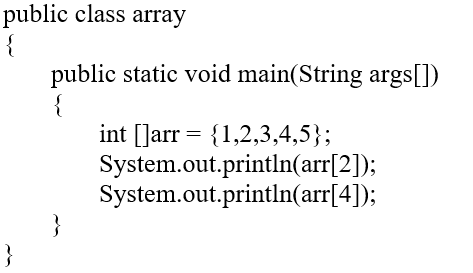What is the output of the following piece of code?

a) 3 and 5
b) 5 and 3
c) 2 and 4
d) 4 and 2

a) 3 and 5

Explanation: Array indexing starts from 0.

5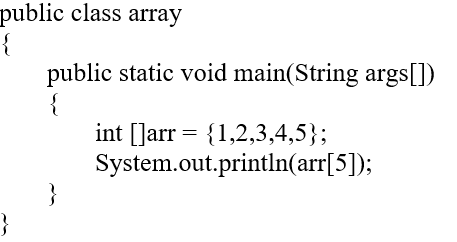What is the output of the following piece of code?

a) 4
b) 5
c) ArrayIndexOutOfBoundsException
d) InvalidInputException

c) ArrayIndexOutOfBoundsException

Explanation: Trying to access an element beyond the limits of an array gives ArrayIndexOutOfBoundsException.

6

When does the ArrayIndexOutOfBoundsException occur?
a) Compile-time
b) Run-time
c) Not an error
d) None of the mentioned

b) Run-time

Explanation: ArrayIndexOutOfBoundsException is a run-time exception and the compilation is error-free.

7

What are the advantages of arrays?
a) Easier to store elements of same data type
b) Used to implement other data structures like stack and queue
c) Convenient way to represent matrices as a 2D array
d) All of the mentioned

d) All of the memtioned

Explanation: Arrays are simple to implement when it comes to matrices of fixed size and type, or to implement other data structures.

8

What are the disadvantages of arrays?
a) We must know beforehand how many elements will be there in the array
b) There are chances of wastage of memory space if elements inserted in an array are lesser than the allocated size
c) Insertion and deletion becomes tedious
d) All of the mentioned

d) All of the mentioned

Explanation: Arrays are of fixed size, hence during the compile time we should know its size and type, since arrays are stored in contiguous locations, insertion and deletion becomes time consuming.

9

Process of inserting an element in stack is called ____________
a) Create
b) Push
c) Evaluation
d) Pop

b) Push

Explanation: Self Explanatory

10

Process of removing an element from stack is called __________
a) Create
b) Push
c) Evaluation
d) Pop

d) Pop

Explanation: Self Explanatory

11

In a stack, if a user tries to remove an element from empty stack it is called _________
a) Underflow
b) Empty collection
c) Overflow
d) Garbage Collection

a) Underflow

Explanation: Self Explanatory

12

Pushing an element into stack already having five elements and stack size of 5, then stack becomes
a) Overflow
b) Crash
c) Underflow
d) User flow

a) Overflow

Explanation: Self Explanatory

13

Entries in a stack are “ordered”. What is the meaning of this statement?
a) A collection of stacks is sortable.
b) Stack entries may be compared with the ‘<‘ operation.
c) The entries are stored in a linked list.
d) There is a Sequential entry that is one by one.

d) There is a Sequential entry that is one by one

Explanation: Self Explanatory

14

Which of the following applications may use a stack?
a) A parentheses balancing program.
b) Tracking of local variables at run time.
c) Compiler Syntax Analyzer.
d) All of the mentioned

d) All of the mentioned

15

Which of the following is not a disadvantage to the usage of array?
a) Fixed size
b) You know the size of the array prior to allocation
c) Insertion based on position
d) Accessing elements at specified positions

d) Accessing elements at specified positions

Explanation: Array elements can be accessed in two steps. First, multiply the size of the data type with the specified position, second, add this value to the base address. Both of these operations can be done in constant time, hence accessing elements at a given index/position is faster.

16

What is the time complexity of inserting at the end in dynamic arrays?
a) O(1)
b) O(n)
c) O(logn)
d) Either O(1) or O(n)

d) Either O(1) or O(n)

Explanation: Depending on whether the array is full or not, the complexity in dynamic array varies. If you try to insert into an array which is not full, then the element is simply stored at the end, this takes O(1) time. If you try to insert into an array which is full, first you will have to allocate an array with double the size of the current array and then copy all the elements into it and finally insert the new element, this takes O(n) time.

17

What is the time complexity to count the number of elements in the linked list?
a) O(1)
b) O(n)
c) O(logn)
d) None of the mentioned

b) O(n)

Explanation: To count the number of elements, you have to traverse through the entire list, hence complexity is O(n).

18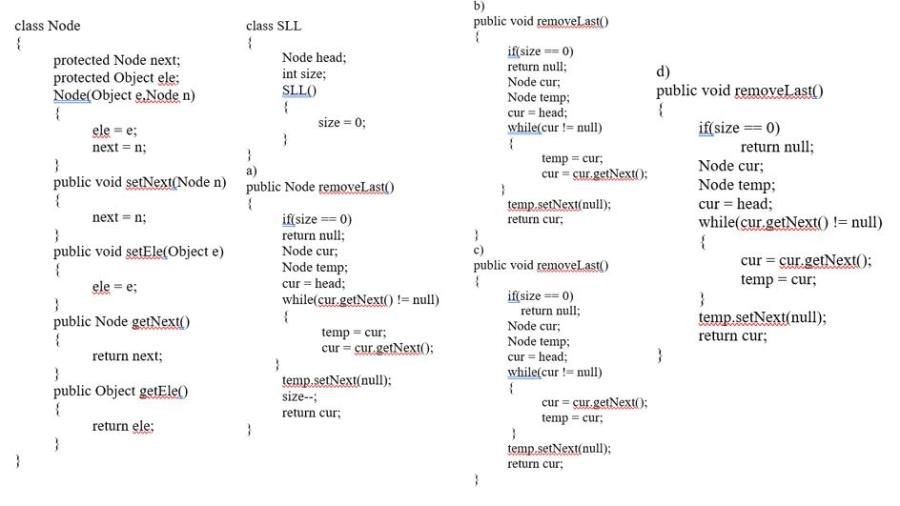Which of the following performs deletion of the last element in the list? Given below is the Node class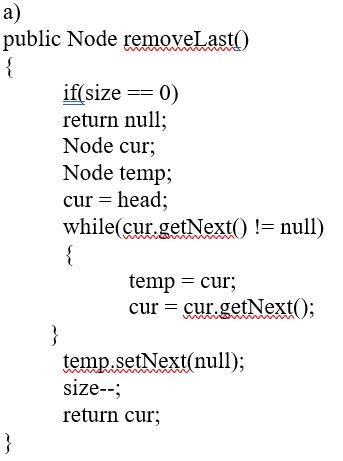Explanation: Since you have to traverse to the end of the list and delete the last node, you need two reference pointers. ‘cur’ to traverse all the way and find the last node, and ‘temp’ is a trailing pointer to ‘cur’. Once you reach the end of the list, setNext of ‘temp’ to null, ‘cur’ is not being pointed to by any node, and hence it is available for garbage collection.

19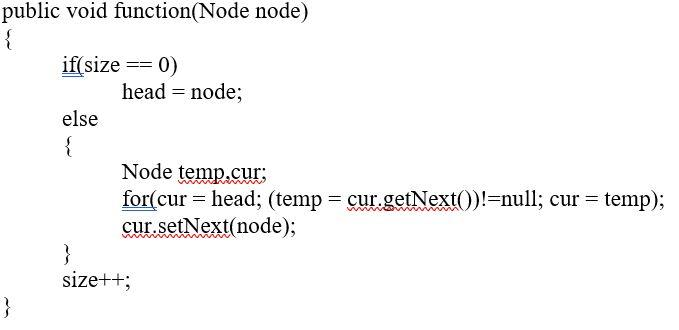What is the functionality of the following code?

a) Inserting a node at the beginning of the list
b) Deleting a node at the beginning of the list
c) Inserting a node at the end of the list
d) Deleting a node at the end of the list

c) Inserting a node at the end of the list

Explanation: The for loop traverses through the list and then inserts a new node as cur.setNext(node);

20

What is the space complexity for deleting a linked list?
a) O(1)
b) O(n)
c) Either O(1) or O(n)
d) O(logn)

a) O(1)

Explanation: You need a temp variable to keep track of current node, hence the space complexity is O(1).

21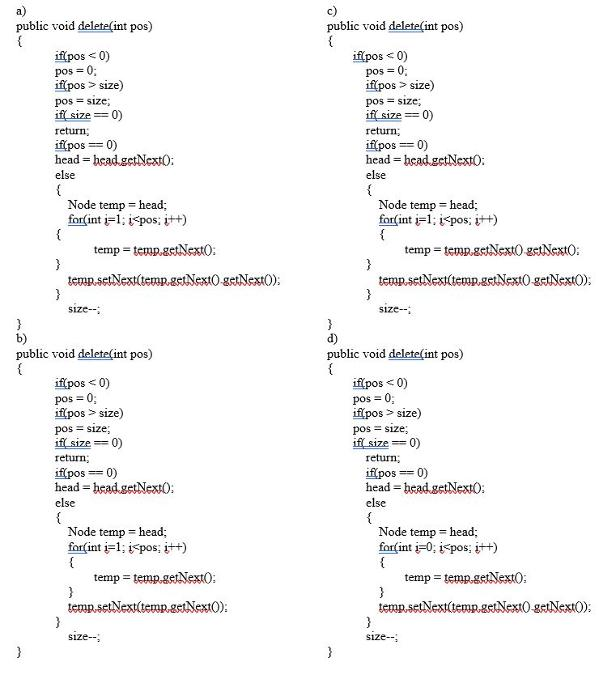How would you delete a node in the singly linked list? The position to be deleted is given.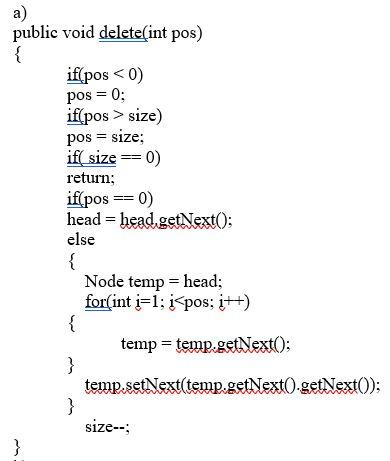Explanation: Loop through the list to get into position one behind the actual position given. temp.setNext(temp.getNext().getNext()) will delete the specified node.

22

Which of these is an application of linked lists?
a) To implement file systems
b) For separate chaining in hash-tables
c) To implement non-binary trees
d) All of the mentioned

d) All of the mentioned

23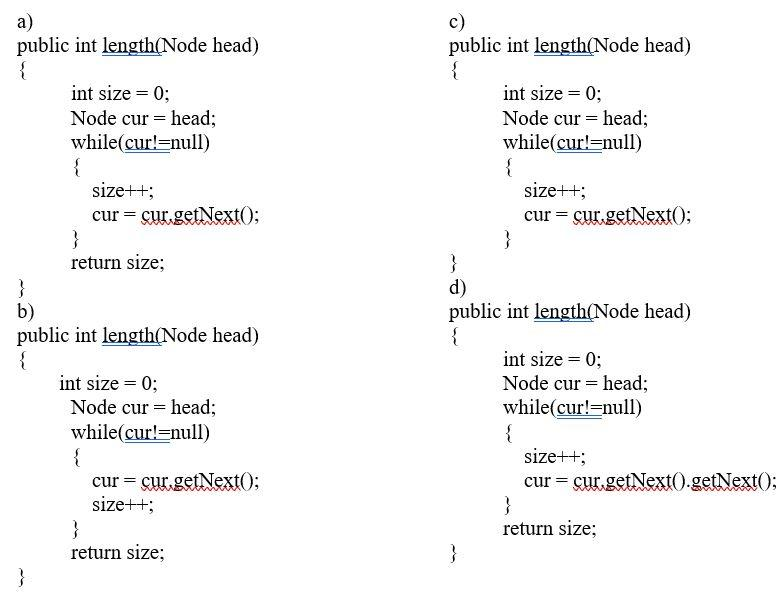Which of the following piece of code has the functionality of counting the number of elements in the list?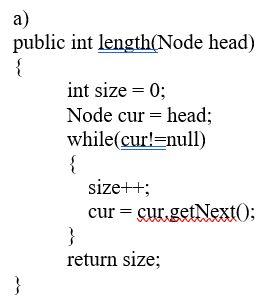Explanation: ‘cur’ pointer traverses through list and increments the size variable until the end of list is reached.

24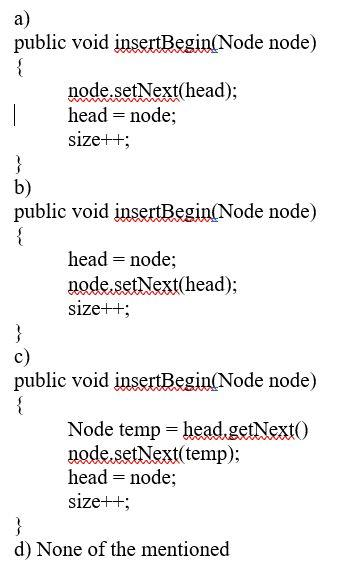How do you insert an element at the beginning of the list?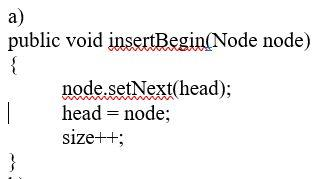Explanation: Set the ‘next’ pointer point to the head of the list and then make this new node as the head.

25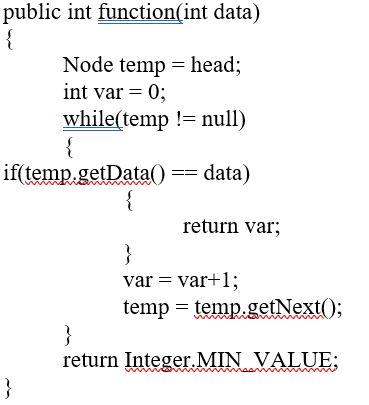What is the functionality of the following piece of code?

a) Find and delete a given element in the list
b) Find and return the given element in the list
c) Find and return the position of the given element in the list
d) Find and insert a new element in the list

c) Find and return the position of the given element in the list

Explanation: When temp is equal to data, the position of data is returned.

26

a) Every node has a successor
b) Time complexity of inserting a new node at the head of the list is O(1)
c) Time complexity for deleting the last node is O(n)
d) None of the mentioned

b) Time complexity of inserting a new node at the head of the list is O(1)

Explanation: Time complexity of inserting a new node at the head of the list is O(n) because you have to traverse through the list to find the tail node.

27

Consider a small circular linked list. How to detect the presence of cycles in this list effectively?
a) Keep one node as head and traverse another temp node till the end to check if its ‘next points to head
b) Have fast and slow pointers with the fast pointer advancing two nodes at a time and slow pointer advancing by one node at a time
c) Cannot determine, you have to pre-define if the list contains cycles
d) None of the mentioned

b) Have fast and slow pointers with the fast pointer advancing two nodes at a time and slow pointer advancing by one node at a time

28

a) You cannot have the ‘next’ pointer point to null in a circular linked list
b) It is faster to traverse the circular linked list
c) You may or may not have the ‘next’ pointer point to null in a circular linked list
d) All of the mentioned

c) You may or may not have the 'next' pointer point to null in a circular linked list

Explanation: The ‘next’ pointer points to null only when the list is empty, otherwise it points to the head of the list.

29

The data structure required for Breadth First Traversal on a graph is?
a) Stack
b) Array
c) Queue
d) Tree

c) Queue

30

A queue is a?
a) FIFO (First In First Out) list
b) LIFO (Last In First Out) list
c) Ordered array
d) Linear tree

a) FIFO (First In First Out) list

31

In Breadth First Search of Graph, which of the following data structure is used?
a) Stack
b) Queue
d) None of the mentioned

b) Queue

32

If the elements “A”, “B”, “C” and “D” are placed in a queue and are deleted one at a time, in what order will they be removed?
a) ABCD
b) DCBA
c) DCAB
d) ABCD

a) ABCD

Explanation: Queue follows FIFO approach.

33

A data structure in which elements can be inserted or deleted at/from both the ends but not in the middle is?
a) Queue
b) Circular queue
c) Dequeue
d) Priority queue

c) Dequeue

34

A normal queue, if implemented using an array of size MAX_SIZE, gets full when
a) Rear = MAX_SIZE – 1
b) Front = (rear + 1)mod MAX_SIZE
c) Front = rear + 1
d) Rear = front

a) Rear = MAX-SIZE - 1

35

Queues serve major role in
a) Simulation of recursion
b) Simulation of arbitrary linked list
c) Simulation of limited resource allocation
d) All of the mentioned

c) Simulation of limited resource allocation

36

A linear list of elements in which deletion can be done from one end (front) and insertion can take place only at the other end (rear) is known as a?
a) Queue
b) Stack
c) Tree

a) Queue

37

What is an AVL tree?

a) a tree which is balanced and is a height balanced tree

b) a tree which is unbalanced and is a height balanced tree

c) a tree with three children

d) a tree with at most 3 children

a) a tree which is balanced and is a height balanced tree

38

Why we need to a binary tree which is height balanced?

a) to avoid formation of skew trees

b) to save memory

c) to attain faster memory access

d) to simplify storing

a) to avoid formation of skew trees